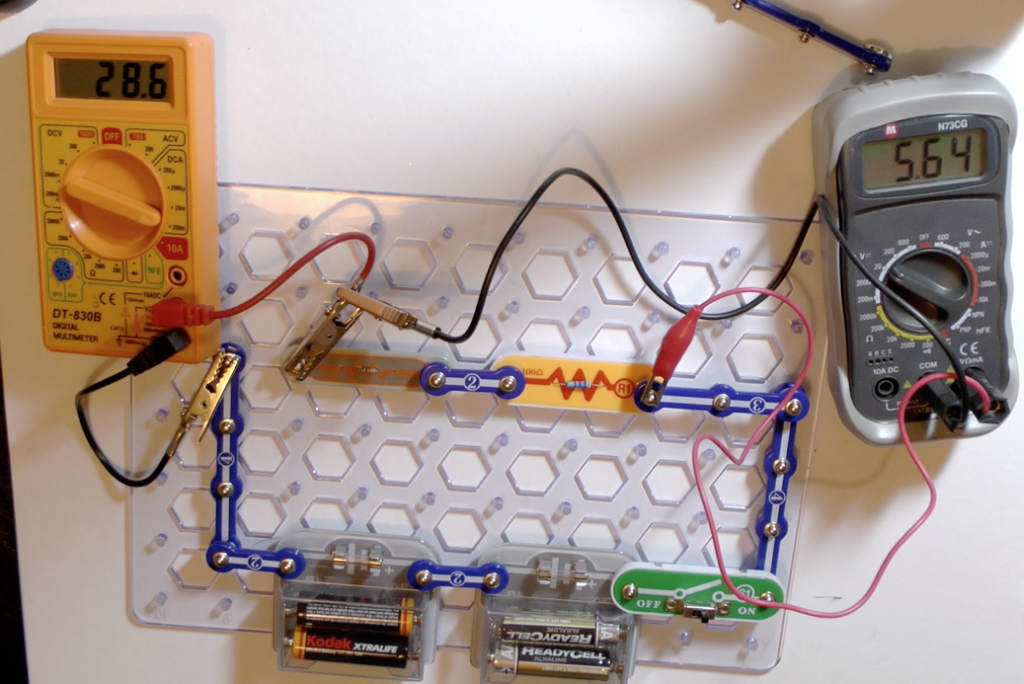### Resistors in series

When resistors are connected in series that means that they are attached end to end and the same electric current flows through all of them. Although they all passed the same current the potential difference, that is the voltage, across each of the resistors is likely to be different.

The photograph shows measurement of the total of two resistors connected in series.Measuring the total of two resistors

Resistance is calculated from the potential difference and the current. The resistance equals potential difference divided by current.